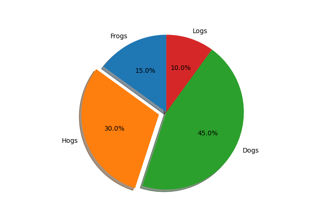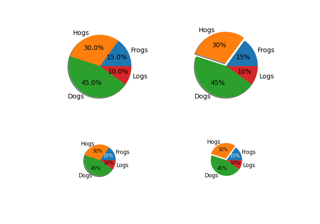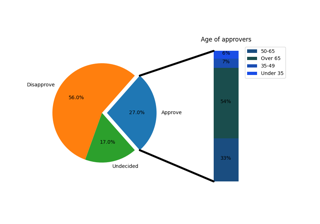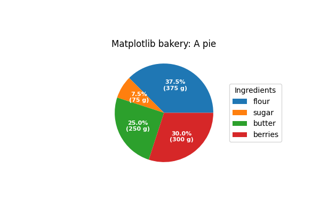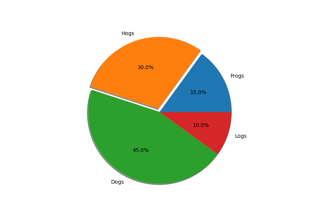# matplotlib.axes.Axes.pie¶

Axes.pie(self, x, explode=None, labels=None, colors=None, autopct=None, pctdistance=0.6, shadow=False, labeldistance=1.1, startangle=0, radius=1, counterclock=True, wedgeprops=None, textprops=None, center=(0, 0), frame=False, rotatelabels=False, *, normalize=None, data=None)[source]

Plot a pie chart.

Make a pie chart of array x. The fractional area of each wedge is given by x/sum(x). If sum(x) < 1, then the values of x give the fractional area directly and the array will not be normalized. The resulting pie will have an empty wedge of size 1 - sum(x).

The wedges are plotted counterclockwise, by default starting from the x-axis.

Parameters: x1D array-likeThe wedge sizes. explodearray-like, default: NoneIf not None, is a len(x) array which specifies the fraction of the radius with which to offset each wedge. labelslist, default: NoneA sequence of strings providing the labels for each wedge colorsarray-like, default: NoneA sequence of colors through which the pie chart will cycle. If None, will use the colors in the currently active cycle. autopctNone or str or callable, default: NoneIf not None, is a string or function used to label the wedges with their numeric value. The label will be placed inside the wedge. If it is a format string, the label will be fmt % pct. If it is a function, it will be called. pctdistancefloat, default: 0.6The ratio between the center of each pie slice and the start of the text generated by autopct. Ignored if autopct is None. shadowbool, default: FalseDraw a shadow beneath the pie. normalize: None or bool, default: NoneWhen True, always make a full pie by normalizing x so that sum(x) == 1. False makes a partial pie if sum(x) <= 1 and raises a ValueError for sum(x) > 1. When None, defaults to True if sum(x) >= 1 and False if sum(x) < 1. Please note that the previous default value of None is now deprecated, and the default will change to True in the next release. Please pass normalize=False explicitly if you want to draw a partial pie. labeldistancefloat or None, default: 1.1The radial distance at which the pie labels are drawn. If set to None, label are not drawn, but are stored for use in legend() startanglefloat, default: 0 degreesThe angle by which the start of the pie is rotated, counterclockwise from the x-axis. radiusfloat, default: 1The radius of the pie. counterclockbool, default: TrueSpecify fractions direction, clockwise or counterclockwise. wedgepropsdict, default: NoneDict of arguments passed to the wedge objects making the pie. For example, you can pass in wedgeprops = {'linewidth': 3} to set the width of the wedge border lines equal to 3. For more details, look at the doc/arguments of the wedge object. By default clip_on=False. textpropsdict, default: NoneDict of arguments to pass to the text objects. center(float, float), default: (0, 0)The coordinates of the center of the chart. framebool, default: FalsePlot axes frame with the chart if true. rotatelabelsbool, default: FalseRotate each label to the angle of the corresponding slice if true. patcheslistA sequence of matplotlib.patches.Wedge instances textslistA list of the label Text instances. autotextslistA list of Text instances for the numeric labels. This will only be returned if the parameter autopct is not None.

Notes

The pie chart will probably look best if the figure and axes are square, or the Axes aspect is equal. This method sets the aspect ratio of the axis to "equal". The axes aspect ratio can be controlled with Axes.set_aspect.

Note

In addition to the above described arguments, this function can take a data keyword argument. If such a data argument is given, the following arguments can also be string s, which is interpreted as data[s] (unless this raises an exception): x, explode, labels, colors.

Objects passed as data must support item access (data[s]) and membership test (s in data).

## Examples using matplotlib.axes.Axes.pie¶